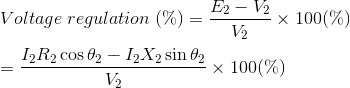# Voltage Regulation - MBT Transformer

Voltage regulation is defined as a measure of change in the voltage magnitude between the sending and receiving end of a component. It is commonly used in power engineering to describe the percentage voltage difference between no-load and full load voltages distribution lines, transmission lines, and transformers.

## Explanation of Voltage Regulation of Transformer

Say an electrical power transformer is open-circuited, meaning that the load is not connected to the secondary terminals. In this situation, the secondary terminal voltage of the transformer will be its secondary induced emf E2.

Whenever a full load is connected to the secondary terminals of the transformer, rated current I2 flows through the secondary circuit, and voltage drop comes into the picture. In this situation, the primary winding will also draw equivalent full load current from the source. The voltage drop in the secondary is I2Z2 where Z2 is the secondary impedance of the transformer.

Now if at this loading condition, anyone measures the voltage between secondary terminals, he or she will get voltage V2 across load terminals which is obviously less than no-load secondary voltage E2 and this is because of the I2Z2 voltage drop in the transformer.

## Expression of Voltage Regulation of Transformer

The equation for the voltage regulation of transformer, represented in percentage, is## Voltage Regulation of Transformer for Lagging Power Factor

Now we will derive the expression of voltage regulation in detail. Say the lagging power factor of the load is cosθ2, which means the angle between secondary current and voltage is θ2.Here, from the above diagram,The angle between OC and OD may be very small, so it can be neglected and OD is considered nearly equal to OC i.e.Voltage regulation of transformer at lagging power factor,## Voltage Regulation of Transformer for Leading Power Factor

Let’s derive the expression of voltage regulation with leading current, say leading power factor of the load is cosθ2, that means the angle between secondary current and voltage is θ2.Here, from the above diagram,The angle between OC and OD may be very small, so it can be neglected and OD is considered nearly equal to OC i.e.Voltage regulation of transformer at leading power factor,## Zero Voltage Regulation of A Transformer

‘Zero voltage regulation’ indicates that there is no difference between its ‘no-load voltage’ and its ‘full-load voltage’. This means that in the voltage regulation equation above, voltage regulation is equal to zero. This is not practical – and is only theoretically possible in the case of an ideal transformer.

Source: Electrical4u

.(+84) 0913.006.538
Skype, Telegram, Wechat ...